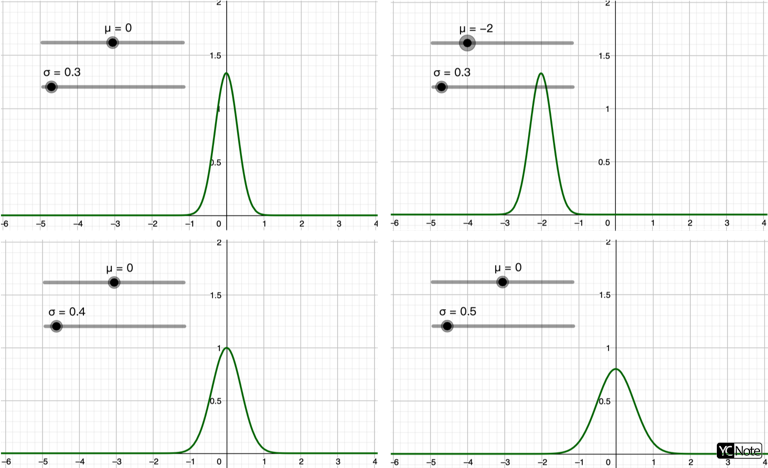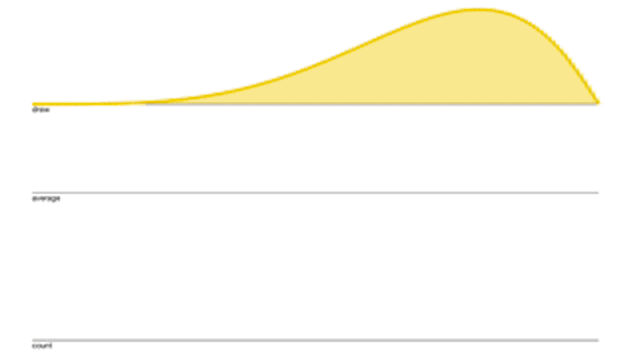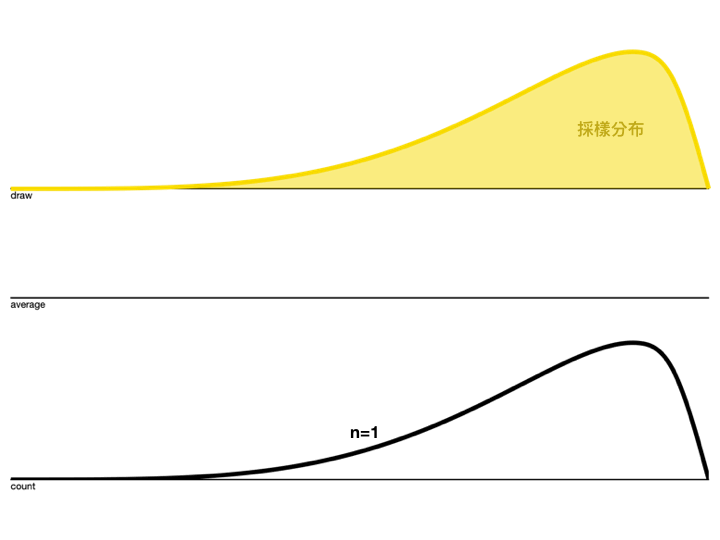# 剖析深度學習 (1)：為什麼Normal Distribution這麼好用？

Posted on February 18, 2020 in AI.ML. View: 12,050

• 為什麼Normal Distribution通常作為雜訊的分布？
• 為何在DL（deep learning），參數的初始化要用Normal Distribution？
• 為何在Bayesian公式裡常常會使用Normal Distribution當作Prior Probability？Courtesy Nas Mouti

### 認識Normal Distribution

$$p_{normal}(x)=\frac{1}{\sqrt{2\pi}\sigma}exp\{{-\frac{1}{2\sigma^2}(x-\mu)^2}\} \ \ ↪︎【1】$$

$$E[g(x)]\equiv\int^{\infty}_{-\infty}g(x)\cdot p(x)dx \ \ ↪︎【2】$$

$$E[g]\equiv \sum_{i}g_i\cdot p_i \ \ ↪︎【3】$$

$$E[g]= \frac{1}{N}\sum_{i=1}^{N}g_i \ \ ↪︎【4】$$

Mean的定義為

$$E[x]\equiv\int^{\infty}_{-\infty}x\cdot p(x)dx \ \ ↪︎【5】$$

Variance的定義為

$$Var[x]\equiv E[(x-E[x])^2] \ \ ↪︎【6】$$

$$Var[x]=E[x^2]-E[x]^2 \ \ ↪︎【7】$$

$$\int^{\infty}_{-\infty}exp\{-a(x+b)^2\}dx=\sqrt{\frac{\pi}{a}} \ \ ↪︎【8】$$

$$\int^{\infty}_{-\infty}p_{normal}(x)dx=\frac{1}{\sqrt{2\pi}\sigma}\int^{\infty}_{-\infty}exp\{{-\frac{1}{2\sigma^2}(x-\mu)^2}\}dx$$

$$\int^{\infty}_{-\infty}p_{normal}(x)dx=\frac{1}{\sqrt{2\pi}\sigma}\sqrt{\frac{\pi}{\frac{1}{2\sigma^2}}}=1 \ \ ↪︎【9】$$

$$E_{x\sim normal}[x]=\int^{\infty}_{-\infty}x\cdot p_{normal}(x)dx=\frac{1}{\sqrt{2\pi}\sigma}\int^{\infty}_{-\infty}x\cdot exp\{{-\frac{1}{2\sigma^2}(x-\mu)^2}\}dx$$

$$s=x-\mu$$ 代入上式，得

$$=\frac{1}{\sqrt{2\pi}\sigma}\int^{\infty}_{-\infty}(s+\mu)\cdot exp\{{-\frac{1}{2\sigma^2}s^2}\}ds$$
$$=\frac{1}{\sqrt{2\pi}\sigma}[\int^{\infty}_{-\infty}s\cdot exp\{{-\frac{1}{2\sigma^2}s^2}\}ds+\int^{\infty}_{-\infty}\mu\cdot exp\{{-\frac{1}{2\sigma^2}s^2}\}ds]$$

$$=\mu\int^{\infty}_{-\infty} \frac{1}{\sqrt{2\pi}\sigma}exp\{{-\frac{1}{2\sigma^2}(x-\mu)^2}\}dx=\mu \int^{\infty}_{-\infty}p_{normal}(x)dx$$

$$E_{x\sim normal}[x]=\mu \ \ ↪︎【10】$$

$$Var_{x\sim normal}[x]=E_{x\sim normal}[x^2]-E_{x\sim normal}[x]^2 \ \ ↪︎【11】$$

$$E_{x\sim normal}[x^2]=\int^{\infty}_{-\infty}x^2p_{normal}(x)dx=\frac{1}{\sqrt{2\pi}\sigma}\int^{\infty}_{-\infty}x^2\cdot exp\{{-\frac{1}{2\sigma^2}(x-\mu)^2}\}dx$$

$$a=\frac{1}{2\sigma^2}$$$$s=x -\mu$$

$$=\sqrt{\frac{a}{\pi}}\int^{\infty}_{-\infty}(s+\mu)^2\cdot exp\{{-a \cdot s^2}\}ds$$

$$=\sqrt{\frac{a}{\pi}}[\int^{\infty}_{-\infty}s^2\cdot exp\{{-a \cdot s^2}\}ds+\int^{\infty}_{-\infty}2s\mu\cdot exp\{{-a \cdot s^2}\}ds+\int^{\infty}_{-\infty}\mu^2\cdot exp\{{-a \cdot s^2}\}ds]$$

$$E_{x\sim normal}[x^2]=\sqrt{\frac{a}{\pi}}\int^{\infty}_{-\infty}s^2\cdot exp\{{-a \cdot s^2}\}ds+\mu^2$$

$$=\sqrt{\frac{a}{\pi}}\frac{-\partial}{\partial a}(\int^{\infty}_{-\infty} exp\{{-a \cdot s^2}\}ds)+\mu^2=\sqrt{\frac{a}{\pi}}\frac{-\partial}{\partial a}(\sqrt{\frac{\pi}{a}})+\mu^2=\frac{1}{2a}+\mu^2$$

$$E_{x\sim normal}[x^2]=\sigma^2+\mu^2 \ \ ↪︎【12】$$

$$Var_{x\sim normal}[x]=\sigma^2+\mu^2-(\mu)^2=\sigma^2 \ \ ↪︎【13】$$### 隨機誤差大都呈現Normal Distribution

Central Limit Theorm:

Let $$\{x_1,x_2,...,x_n\}$$ be a random sample of size $$n$$ — that is, a sequence of independent and identically distributed (i.i.d.) random variables drawn from a distribution of expected value $$E[x_i]=\mu$$ and variance $$Var[x_i]=\sigma^2<\infty$$. Suppose we are interested in the sample average: $$S_n=(x_1+x_2+...+x_n)/n$$ , Then as $$n\rightarrow \infty$$ , $$S_n$$ follows normal distribution $$p_{normal}(\mu,(\frac{\sigma}{\sqrt{n}})^2)$$.

$$E[S_n]=\mu \ \ ↪︎【14】$$
$$Var[S_n]=\frac{\sigma^2}{n} \ \ ↪︎【15】$$

$$n=1$$時，$$S_n$$的分布其實就是 $$p(x)$$ 的分布，當然Mean和Variance會和原分布 $$p(x)$$ 一模一樣。### Normal Distribution是所有機率分布當中假設最少的

First, many distributions we wish to model are truly close to being normal distributions. The central limit theorem shows that the sum of many independent random variables is approximately normally distributed. This means that in practice, many complicated systems can be modeled successfully as normally distributed noise, even if the system can be decomposed into parts with more structured behavior.

Second, out of all possible probability distributions with the same variance, the normal distribution encodes the maximum amount of uncertainty over the real numbers. We can thus think of the normal distribution as being the one that inserts the least amount of prior knowledge into a model.

-- from: Deep Learning 3.9.3

• Normal Distribution是隨機性最大的分布
• Normal Distribution是最少先驗知識（Prior Knowledge）假設的

$$H\equiv E[-ln\ p(x)] \ \ ↪︎【16】$$

$$H_{x\sim p(x)}=E[-ln\ p(x)]=- \int^{\infty}_{-\infty}ln\ p(x)\cdot p(x)dx \ \ ↪︎【17】$$

$$H_{x\sim normal}=- \int^{\infty}_{-\infty}p_{normal}(x)\cdot ln\ p_{normal}(x) dx$$
$$=- \int^{\infty}_{-\infty}\frac{1}{\sqrt{2\pi}\sigma}exp\{{-\frac{1}{2\sigma^2}(x-\mu)^2}\} \cdot [ln(\frac{1}{\sqrt{2\pi}\sigma})-\frac{1}{2\sigma^2}(x-\mu)^2]dx$$
$$=ln(\sqrt{2\pi}\sigma)+ \frac{1}{2\sigma^2} \int^{\infty}_{-\infty}\frac{1}{\sqrt{2\pi}\sigma}exp\{{-\frac{1}{2\sigma^2}(x-\mu)^2}\} \cdot (x-\mu)^2dx$$
$$=ln(\sqrt{2\pi}\sigma)+ \frac{1}{2\sigma^2} E_{x\sim normal}[(x-\mu)^2]$$
$$=ln(\sqrt{2\pi}\sigma)+ \frac{1}{2\sigma^2} Var_{x\sim normal}[x]$$
$$H_{x\sim normal}=ln(\sqrt{2\pi}\sigma)+\frac{1}{2}=\frac{1}{2}ln(2\pi e\sigma^2) \ \ ↪︎【18】$$

• $$\int p(x)dx=1 \ \ ↪︎【19】$$
• $$E[x]=\mu \ \ ↪︎【20】$$
• $$Var[x]=\sigma^2 \ \ ↪︎【21】$$

$$p^*(x) = argmax_{p(x)}\ H_{x\sim p(x)}=argmin_{p(x)}\int^{\infty}_{-\infty}ln\ p(x)\cdot p(x)dx \ \ ↪︎【22】$$

$$L=\int^{\infty}_{-\infty}ln\ p(x)\cdot p(x)dx-\lambda_1 (\int^{\infty}_{-\infty}p(x)dx-1)-\lambda_2(\int^{\infty}_{-\infty}x\cdot p(x)dx-\mu)-\lambda_3(\int^{\infty}_{-\infty}(x-\mu)^2\cdot p(x)dx-\sigma^2)$$
$$=\int^{\infty}_{-\infty}[-\lambda_1 p(x)-\lambda_2 p(x)x-\lambda_3 p(x)(x-\mu)^2+p(x)ln(p(x))]dx+\lambda_1 +\mu\lambda_2+\sigma^2\lambda_3 \ \ ↪︎【23】$$

$$0=\frac{\partial L}{\partial p(x)}|_{p^*(x)}=\int^{\infty}_{-\infty}[-\lambda_1-\lambda_2 x-\lambda_3 (x-\mu)^2+ln(p^*(x))+1]dx \ \ ↪︎【24】$$

$$p^*(x)=exp\{\lambda_1+\lambda_2 x+\lambda_3 (x-\mu)^2-1\} \ \ ↪︎【25】$$

$$\lambda_1=1-ln(\sqrt{2\pi}\sigma);\ \lambda_2=0;\ \lambda_3=-\frac{1}{2\sigma^2} \ \ ↪︎【26】$$

### Back to the Question

• 中央極限定理告訴我們：如果今天採樣數量 $$n$$ 增加到一定的量，$$S_n$$的分布會趨近於Normal Distribution
• 自然界的巨觀現象往往是源自於微觀現象的累積，我們量測的物理量常常來自於多個微小貢獻疊加而成，而不管這些微小貢獻本身的分布狀況如何，其巨觀的物理量因為中央極限定理而成為Normal Distribution，這也是為什麼「隨機誤差大都呈現Normal Distribution」的原因
• Entropy是衡量隨機性的指標，定義為：$$H\equiv E[-ln\ p(x)]$$
• 在給定Mean和Variance下，Normal Distribution為所有分布當中Entropy最大的。這也同時意味著，Normal Distribution是隨機性最大的，Normal Distribution是額外假設最少的

• 如果沒有特別理由，請假設隨機誤差為Normal Distribution，因為自然界的隨機誤差大都呈現Normal Distribution
• 如果想要人為假設一個分布，請優先選擇Normal Distribution，因為它是包含最少先驗知識（Prior Knowledge）的分布

• Q：為什麼Normal Distribution通常作為雜訊的分布？
• A：因為自然界的隨機誤差大都呈現Normal Distribution
• Q：為何在DL（deep learning），參數的初始化要用Normal Distribution？
• A：因為它是包含最少先驗知識（Prior Knowledge）的分布
• Q：為何在Bayesian公式裡常常會使用Normal Distribution當作Prior Probability？
• A：因為它是包含最少先驗知識（Prior Knowledge）的分布